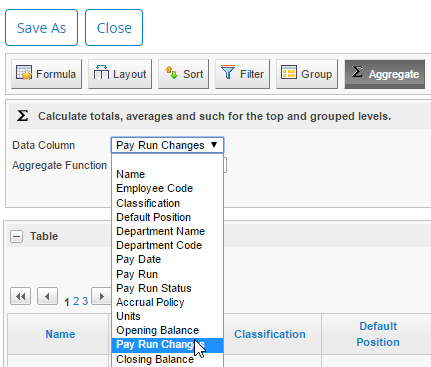## Functions - Aggregate

Once you have run a report and want a column of information to do calculations for you, you will need help from the Aggregate selection.

Choose the column that you want to do a calculation for from the "Data Column" drop down list.Next, we have to select what calculation we want done to the Pay Run Changes.Average: calculates the average of the values

Standard Deviation: shows how much variation there is between the values and the average of the values

Count: the total number of values (in this example, how many pay runs)

Distinct Count: total number of unique values

Minimum: the lowest value

Maximum: the highest value

After selecting "Sum", there will now be a grand total of the pay run changes listed.